# 2nd PUC Basic Maths Previous Year Question Paper June 2016

Students can Download 2nd PUC Basic Maths Previous Year Question Paper June 2016, Karnataka 2nd PUC Maths Model Question Papers with Answers helps you to revise the complete Karnataka State Board Syllabus and score more marks in your examinations.

## Karnataka 2nd PUC Basic Maths Previous Year Question Paper June 2016

Time: 3.15 hours
Max Marks: 100

Instructions:

• The question paper has 5 parts A, B, C, D and E. Answer al the parts
• Part-A carries 10 marks\, Parts – B carries 20 marks, Part – C carries 3 marks, Part – D carries 30 marks and Part – E carries 1 marks.
• Write the question number properly as indicated in the question paper.

Part – A

Answer All the ten questions: (10 × 1 = 10)

Question 1.
Find x such that $$\left[ \begin{matrix} 3 & x \\ 4 & 7 \end{matrix} \right]$$ is symmetric
X = 4

Question 2.
Find n if nC8 = nC7
nC8 = nC7nC8 = nC7 ⇒ 8 = n – 7 ⇒ n = 15

Question 3.
Negate the proposition “4 is an even integer or 7 is a prime number”.
4 is not an even integer and 7 is not a prime number.

Question 4.
Find the value of x if 32 : x = 75 : 50.
$$x=\frac{32 \times 50}{75}=\frac{64}{3}$$

Question 5.
Find the income obtained by investing ₹ 3,600 in 5% stock at 90.
Income = $$\frac { 5 }{ 100 }$$ × 3600 = 180Question 6.
Find the value of 3 sin 10° – 4 sin 10°.
3 sin 10° – 4 sin 10° = sin 3.10° = sin 30° = $$\frac { 1 }{ 2 }$$

Question 7.
If x2 + y2 – 4x – 8y + k = 0 represents a point circle find k.
R = $$\sqrt{g^{2}+f^{2}-c}$$, g = -2, f = -4,c = k, r = 0.
∴ $$\sqrt{4+16-k}=0$$
20 – k = 0 ⇒ k = 20

Question 8.
Evaluate $$\lim _{x \rightarrow 0}(1+3 x)^{\frac{1}{x}}$$
$$\lim _{x \rightarrow 0}(1+3 x)^{\frac{1}{x}}=e^{3}$$

Question 9.
If y = cos (x3) find $$\frac{\mathrm{d} \mathrm{y}}{\mathrm{d} \mathrm{x}}$$
Let y = Cos(x3)
∴ $$\frac{\mathrm{d} \mathrm{y}}{\mathrm{d} \mathrm{x}}$$ = sin(x3).3x2

Question 10.
Evaluate : ∫(xe + ex – log a)dx
$$\frac{x^{e+1}}{e+1}+e^{x}-\log a \cdot x+C$$

Part – B

Answer any ten questions. (10 × 2 = 20)

Question 11.
Solve using Cramer’r rule:
5x + 7y = 3
7x + 5y = 9
5x + 7y = 3
7x + 5y = 9
Δ = $$\left| \begin{matrix} 5 & 7 \\ 7 & 5 \end{matrix} \right|$$ = 25 – 69 = -24 Δx = $$\left| \begin{matrix} 3 & 7 \\ 9 & 5 \end{matrix} \right|$$ = 15 – 63 = -48 Δy = $$\left| \begin{matrix} 5 & 3 \\ 7 & 9 \end{matrix} \right|$$ = 45 – 21 = 24
∴ x = $$\frac{\Delta_{x}}{\Delta}=\frac{-48}{-24}$$ = 2 y = $$\frac{\Delta_{y}}{\Delta}=\frac{24}{-24}$$ = -1
∴ x = 2, y = -1

Question 12.
Find the number of parallelograms that can be formed form a set of 6 parallel lines intersecting another set of 4 parallel lines.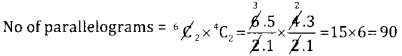Question 13.
What is the probability that there will be 53 Mondays in a randomly selected leap year?
Every year has 52 complete weeks 8 = 364 days (52 × 7) A leap year has 366 days, in the remaining two days we have to see what is the probability I getting Monday, the two days may occur in any one of the following (Sunday, Monday) (Mon,Tue), (Wed, Thur) (Thur,Fri) (Fri, Satur) (Satur, Sun) Out of the 7 Possibilities, the first two cases have Monday
∴ P(53Mondays in a leap) = $$\frac { 2 }{ 7 }$$

Question 14.
Write the converse and income of the statement “If Maths is easy then child is brave”.
Converse = (q → P) = If the child is brave then maths is easy
Inverse = (~ P → ~q) = If maths is not easy then the child is not brave.

Question 15.
Divide 6,000 in the ratio 3 : 4 : 5.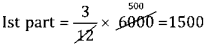II part = $$\frac{4}{12}$$ × 6000 = 2000

III part = $$\frac{5}{12}$$ × 6000 = 2500

Question 16.
A banker pays 2,380 on a bill of 2,500,73 days before the legally due date. Find the rate of discount charged by the banker.
F = ₹ 2,500, discounted value = 2380, t = 73 days, t = $$\frac{73}{365}=\frac{1}{5}$$ yr
Discounted value = F (1 – tr}
2380 = 2500 $$\left(1-\frac{1}{5} \times r\right)$$ ⇒ $$\frac{2380}{2500}$$ = 1 – $$\frac{r}{5}$$ ⇒ 1 – 0.952
0.2r = 0.048
r = $$\frac{0.048}{0.2}$$ = 24 = 24%

Question 17.
If A + B + C = 180° and tan A = 1, tan B=2, show that tan C = 3,
Given A + B + C = 180° ⇒ A + B = 180 – C ∴ tan(A + B) = tan (180 – C)Question 18.
Find the focus and equation of the directrix of the parabol x2 = – 16y
Given x2 = – 16y
Compare x2 = -4ay ⇒ 4a = 16 ⇒ a = 4
The curve turns down words ∴ focus S = (0,-4)
Equation of directrix is y = 4 or y – 4 = 0

Question 19.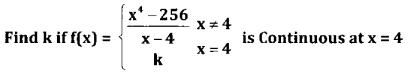Given the function is continuous $$\lim _{x \rightarrow 4}$$ f(x) = f(4)
$$\lim _{x \rightarrow 4} \frac{x^{4}-4^{4}}{x-4}=K$$ ∴ k = 4.4+-1 = 4.43 = 44 = 256Question 20.
If y = xx find $$\frac{d y}{d x}$$
dx
Let y = xx
log y = x.log x
$$\frac{1}{y} \frac{d y}{d x}=x, \frac{1}{x}+\log x, 1 \Rightarrow \frac{d y}{d x}$$ = y (1 + log x) = xx(1 + logx)

Question 21.
When brakes are applied to a moving car, the car travels a distance ‘s’ feet in ‘t’ secs is given by s = 20t – 40t2. When does the car stop?
Given s = 20t – 40t2
v = $$\frac{d s}{d t}$$ = 20 – 80t
The car stops when the velocity = 0 ⇒ 20 – 80t = 0 ⇒ t = $$\frac{1}{4}$$ sec

Question 22.
The radius of a sphere is increasing at the rate of 0.5 mts / sec. Find the rate of Increase of its volume when r=1.5 mts.
Given $$\frac{d r}{d t}$$ = 0.5 mts / sec. $$\frac{d v}{d t}$$ = ? when r =1.5mts v = $$\frac{4}{3}$$πr3Question 23.
Evaluate : $$\int \frac{e^{x}}{e^{x}-1} d x$$
$$\int \frac{e^{x}}{e^{x}-1} d x$$ = log (ex+ 1) + C ∴ $$\int \frac{f^{\prime}(x)}{f(x)}$$ dx = log(f(x))

Question 24.
Evaluate $$\int_{1}^{2}\left(x+e^{x}\right) d x$$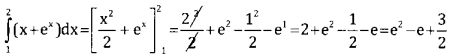Part – C

Answer any ten questions: (10 × 3 = 30)

Question 25.
If A = $$\left[ \begin{matrix} 2 & -1 \\ 1 & 4 \end{matrix} \right]$$ and B = $$\left[ \begin{matrix} -3 & 2 \\ -1 & 4 \end{matrix} \right]$$ show that (AB)’ = B’ A’.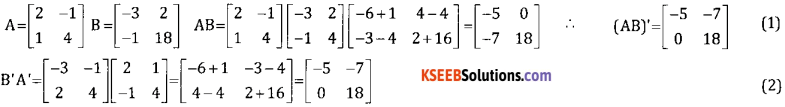From 1 and 2 we get (AB)’ = B’A’

Question 26.
Solve for $$\left[ \begin{matrix} x+2 & 3 & 4 \\ 2 & x+3 & 4 \\ 2 & 3 & x+4 \end{matrix} \right] =\quad 0$$Question 27.
Find the number of permutations of the letters of the word Engineering How many of these
(a) Begin with GRIN
(b) Have all 3 E’s together?
The word has 11 letters, E = 3, N = 3, G = 2, I = 2
Total permutations $$\frac{11 !}{3 !, 3 !, 2 ! 2 !}$$
(a) Permutations word which begin with GRIN = $$\frac{7 !}{3 ! 2 !}$$
(b) All 3E’s are together Consider 3E as one unit remaining letters = 8 + 1 = 9
N = 3,G = 2, 1 – 2 No of permutation = $$\frac{9 !}{3 ! 2 ! 2 !}$$

Question 28.
One Card is randomly drawn from a pack of 52 cards, Find the probability that
(a) Card is either black or jack
(b) Card is red
(c) Card is diamond.
Totally there are 52 cards and any one can be drawn
∴ Total no cases = n = 52

(a) There are 26 black cards and 2 jack totally = 28 cards ∴ no of favourable case = m = 28
∴ P(black or jack) = $$\frac{28}{52}$$

(b) There are 26 red cards
∴ m = 26,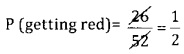(C) There are 13 diamonds cards
∴ m = 13,
P(getting diamond) = $$\frac{13}{52}=\frac{1}{4}$$

Question 29.
Walking 4 kmph a student reaches his college 5 minutes late and if he walks at 5 kmph, he reaches $$2 \frac{1}{2}$$ minutes early. What is the distance from his house to the college?
Let the distance from his house to the college = x km
Time taken to Cover x kns at 4km ph = $$\frac{x}{4}$$ hr5
Time to cover 5 km ph = $$\frac{x}{5}$$ hrsQuestion 30.
A bill for ₹ 12,900 was drawn on 3 Feb, 2014 at 6 months and discounted on 13 March 2014 at 8% p.a. For what sum was the bill discounted ?
F = 12,900, bill period = 6 months, Drawn date = 03 – 2 – 14
∴ LDD = DD + BP + Grace period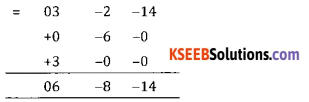Date of discount 13-3-2014. This means, the no of days from 13-2-2014 to 06-08-14 becomes the unexpired period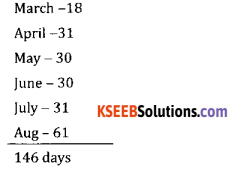∴ t = 145 days = $$\frac{146}{365}$$ year, r = 80% = 0.08, DV = ?
BD = Ftr = 12,900 × $$\frac{146}{365}$$ × 0.08 = 412.8
∴ Discounted value = 12,900 – 412.8 = 12,487.20

Question 31.
Prathik sells out ₹ 6,000 of 7.5% stock at 108 and reinvests the proceeds in 9% stock. If Prathik’s income increase by ₹ 270, at what price did Prathik buy 9% stock?
To calculate income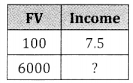Income = $$\frac{6000 \times 7.5}{10}$$ = 459.0 = ₹ 450For 9% stock given income increase by ₹ 270
∴ New income = 450 + 270 = ₹ 270.Question 32.
A furniture dealer sold furniture for ₹ 21,000 and added 5% sales tax to the quoted price. The customers agree to buy it for ₹ 21,000 including sales tax. Find the discount he received.
Discount = 5% 21,00 = $$\frac{5}{10}$$ × 21,000 = 1050

Question 33.
Prove that $$\frac{\cos 75^{\circ}+\cos 15^{\circ}}{\sin 75^{\circ}-\sin 15^{\circ}}=\sqrt{3}$$Question 34.
Find equation of the parabola given that its vertex is (0,0) axis is Y-axis and passed through (-1,-3).
Given v = (0,0) and axis is y-axis
The parabola is of the form x2 = 4ay or x2 = -4ay
But this equation passes through (-1,-0) which is in III qhadrant.
∴ The curve turn down words and is of the form x2 = – 4ay, But it passes through (-1,-3)
∴ (-1)2 = -4a(-3) ⇒ 1 = 12a ⇒ a = $$\frac{1}{12}$$
∴ The required equation is x2 = -4 . $$\frac{1}{12}$$y ⇒ x2 = $$\frac{-1}{3}$$ or 3x2 + y = 0

Question 35.
If x = $$\frac{1-t^{2}}{1+t^{2}}$$ y = $$\frac{2 t}{1+t^{2}}$$ find $$\frac{\mathrm{dy}}{\mathrm{dt}}$$
Differentiate both w.r.ttIf the total cost function is given by C(x) = 350 + 12x + $$\frac{x^{2}}{4}$$ and revenue function is given by R(x) = 75x – 2x2, find the level of output at which profit is maximum.
Profit function = TR – TC = 75x – 2x – 350 – 12x – $$\frac{x^{2}}{4}$$ p(x) = -2x2 – x2 + 63x – 350
For maximum profit differentiate w.r.t x $$\frac{d}{d x}$$ P(x) = 0126 = 9x ⇒ x = $$\frac{126}{9}$$ = 14 ∴ x = 14

Question 37.
Evaluate ∫x2 log xdxQuestion 38.
Evaluate $$\int \frac{1+\cos x}{1-\cos x} d x$$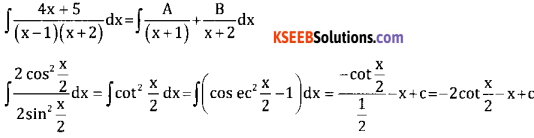Part – D

Answer any six questions: (6 × 5 = 30)

Question 39.
Find the term independent of x in $$\left(x^{3}-\frac{3}{x^{2}}\right)^{15}$$
Tr+1 = nCr xn-r ar
Here x → x3a → $$\frac{-3}{x^{2}}$$ n → 15
Tr+1 = 15Cr (x3)15 – r $$\left(\frac{-3}{x^{2}}\right)$$ = 15Cr . x45 – 3r (-3)r . x-2r
Tr+1 = 15Cr(-3)r . x45 – 5r
To find the term independent of x equate the power of x = 0
⇒ 45 – 5r = 0 ⇒ 45 = 5r = 0 ⇒ r = 9
T9+1 = 15C9(-3)9 . x0 = 15C9(-3)9
T10 = 39, 15C9 is the term independent of x

Question 40.
Resolve into partial fractions $$\frac{3 x+2}{(x-2)(x+3)^{2}}$$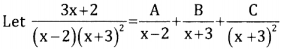3x + 2 = A(x +3 )2 + B(x – 2)(x – 3) + C(x – 2)
Put x = 2,6 + 2 = A (2 + 3))2 ⇒ A = $$\frac { 8 }{ 25 }$$
Put x = -3 -9 + 2 = 0 + 0 + C(-5)
-7 = -5C ⇒ C = 715
Put x = 0 we get B = $$-\frac { 8 }{ 25 }$$Question 41.
Verify whether the proposition ~(p ∨ q) → (~P ∧ ~q) is a tautology contradiction or neither.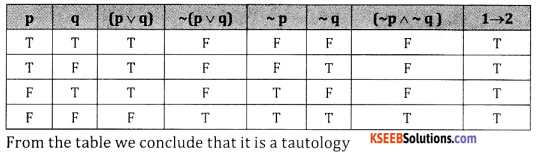Question 42.
Two taps can separately fill a tank in 12 minutes and 15 minutes respectively. The tank when full can be emptied by a drain tap in 20 minutes. When the tank was empty all the three taps were opened simultaneously. In what time will the tank be filled up?
1st tap can fill $$\frac{1}{12}^{\text {th }}$$ tank in 1 min 2nd tap can fill $$\frac{1}{15}^{\text {th }}$$ tank in 1 min
Drain pipe drain out = $$\frac{1}{20}^{\text {th }}$$ tank in 1 min
∴ In 1 min = $$\frac{1}{12}+\frac{1}{15}-\frac{1}{20}=\frac{15+12-9}{180}=\frac{18}{180}=\frac{1}{10}$$ of tank will get filled
∴ The tank will get filled in 10 minutes.

Question 43.
ABC company required 1000 hours to produce first 30 engines. If the learning effect is 90%, find the total labour cost at ₹ 20 per hour to produce total of 120 engines.
Assume 1 lot = 30 engines
∴ 120 engines = 4 lotsThe total no of hrs to produce 4 lots = 3240
The lab cost at 20 per hour = 3240 × 20 = ₹ 64,800

Question 44.
Solve the L.P.P graphically
Maximize Z = 2x + 3y
Subject to constraints x + y ≤ 400, 2x + y ≤ 400, x,y ≥ 0.
Consider
X + y = 400 2x + y = 600Point of intersection of x + y = 400 & 2x + y = 600Function is maximum at the point A (0.400) and maximum value = 1200

Question 45.
The angles of elevation of the top of a tower the base and the top of a building are 60° and 30°. 3x + 5y ≤ 15, 5x + 2y ≤ 10 and x ≥ 0, y ≥ 0.
Let AB = Building & CD is the tower. From the rt angled ∆ ACD
We have tan 60 = $$\frac { h }{ x }$$
√3 = $$\frac { h }{ x }$$ ⇒ x = √3(h -20)
From 1 and 2 wet √3(h – 20) = $$\frac{\mathrm{h}}{\sqrt{3}}$$ ⇒ 3(h – 20) = h ⇒ 3h – 60 = h ⇒ 3h – 60 = h ⇒ 2h = 60 ⇒ h = 30 mts
∴ Height of the tower = 30mts

Question 46.
Find the equation of circle passing through points (5,3) (1,5) (3,-1)
Let the required equation of the circle is
x2 + y2 + 2gx + 2fg + c = 0
But this eqn passes through the points
(5,3) 10g + 6f + C + 34 = 0
(1,5) 2g + 10 f + C + 26 = 0
(3,-1) 6g – 2f + C + 10 = 0
Solving 2,3 and 4 we get
2 – 3 ⇒ 2g – f + 2 = 0
3 – 4 ⇒ g – 3f – 4 = 0
Again solving we get y = -2, f = -2, c = -2
This the required egn of the circle is x2 + y2 – 4x – 4y -2 = 0

Question 47.
If y = $$(x+\sqrt{1+x^{2}})^{m}$$ prove that (1 + x2)y2 + xy1 – m2y=0
Given y = $$(x+\sqrt{1+x^{2}})^{m}$$
Diff. w.r.tx$$\sqrt{1+x^{2}} y_{1}$$ = my S.BS
(1 + x2)y21 = m2y2
Again differentiate w.r.t.x (1 + x2).2y1y2 + y21 (2x) = m2.2yy1 ÷ 2y1 ∴ (1 + x2).y2 + xy1 – m2y = 0Question 48.
Find the area enclosed between parabola y2 = x and the line x + y = 2.
Given y2 = x and x + y = 2
⇒ (2 – x)2 = x ∴ y = 2 – x
⇒ 4 + x2 – 4x – x = 0 ⇒ x2 – 5x – 4 = 0 ⇒ x = 4 orPart – E

Answer any one questions: (1 × 10 = 10)

Question 49.
(a) A school wants to award to award its students for the values of leadership, good behavior and hard work with a total cash award of ₹ 6,000. Three times the award money for hard work added to that given for leadership amounts to 11,000. The award money for leadership and hard work together is double the one given for good behavior. Calculate the award money given for each value, using matrix method.
Let the values of punctuality, good behavior and hard work be denoted by x,y & z respectively
x + y + z = 6000
x + oy + 3z = 11,000
x + z = 2y or x – 2y + z = 0
The given equations in matrix form is $$\left[ \begin{matrix} 1 & 1 & 1 \\ 1 & 0 & 3 \\ 1 & -2 & 1 \end{matrix} \right] \left[ \begin{matrix} x \\ y \\ z \end{matrix} \right] \left[ \begin{matrix} 6000 \\ 11,000 \\ 0 \end{matrix} \right]$$
A x = B
⇒ X = A-1B
Where A = $$\left[ \begin{matrix} 1 & 1 & 1 \\ 1 & 0 & 3 \\ 1 & -2 & 1 \end{matrix} \right]$$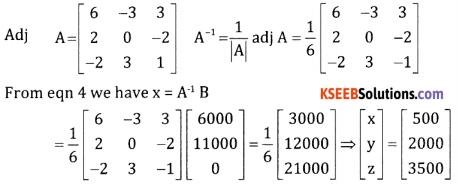X = ₹ 500, y = ₹ 2000, z = ₹ 3500.(b). A company produces two products P and Q. Each P require 4 hours of grinding and 2 hours of polishing and each Q requires 2 hours of grinding and 5 hours of polishing. The total available hours for grinding is 20 and for polishing is 24. Profit per unit of P is 6 and that of Q is ₹ 8. Formulate the L.P.P
Let x be the units of P manufactured and y be the units of Q manufactured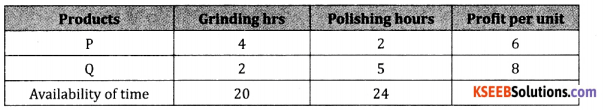Maximize z = 6x + 8y
Subject to constraints

Question 50.
(a). Prove that $$\lim _{\theta \rightarrow 0} \frac{\sin \theta}{\theta}=1$$, where θ is in radius.
Let ‘O’ the centre of unit circle, assume that is measured in positive radians.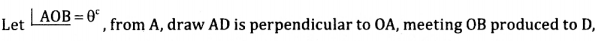From the figure, we have area of ∆AOB = area of sector AOB = area of ∆AOD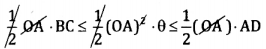∴ Area of sector = $$\frac { 1 }{ 2 }$$ (radius)2 × angle in radians
From the triangle DOA, tan θ = $$\frac{\mathrm{DA}}{\mathrm{OA}}$$ [∴ DA = r tanθ]
From the triangle BOC, sin θ = $$\frac{\mathrm{BC}}{\mathrm{OB}}$$ [∴ BC = r sin θ]
(1) becomes
⇒ BC ≤ θ ≤ DA
⇒ sin θ ≤ θ ≤ tan θDividing by sin θ(b). Find the value of (1.1)5 using binomial theorem upto 4 decimal places.